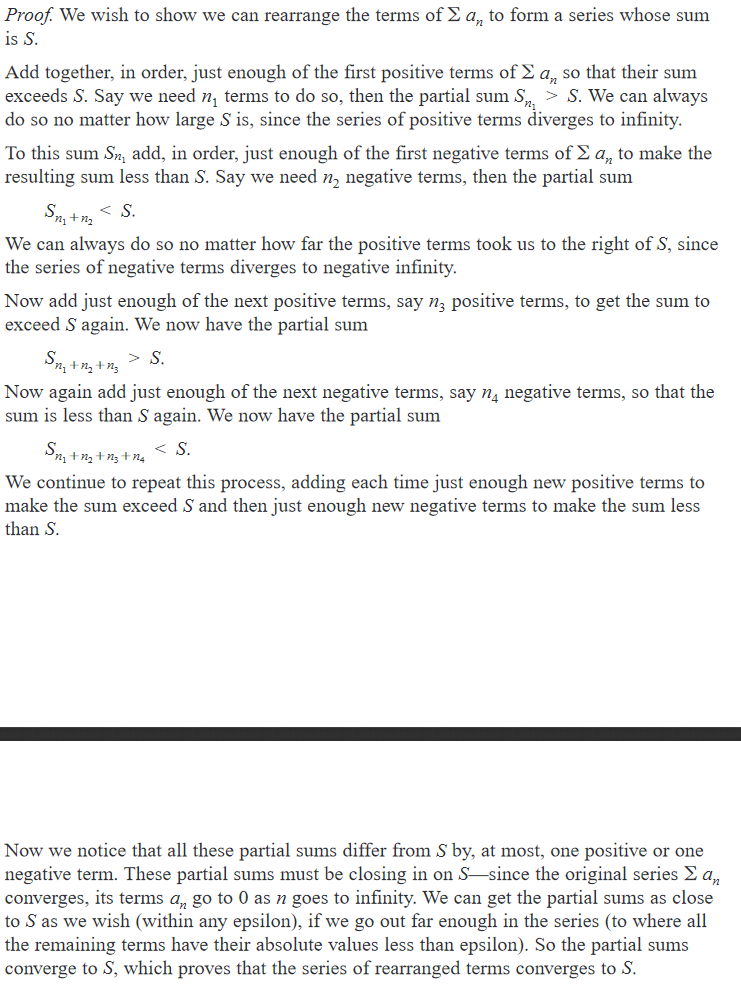Show Question
Math and science::Analysis::Tao::08. Infinite sets

# Bernhard Riemann's rearrangement theorem

Let $$\sum_{n=0}^{\infty} a_n$$ be a series of reals which is conditionally convergent, but not absolutely convergent, and let L be any real number. Then there exists a bijection $$j : \mathbb{N} \rightarrow \mathbb{N}$$ such that $$\sum_{m=0}^{\infty}a_{j(m)}$$ converges conditionally to L.

A supporting lemma can be defined to design the proof:

In a conditionally (but not absolutely) convergent series, both the sum of the positive terms and the sum of the negative terms are divergent series.

An interesting thing to still investigate is how the proof confirms convergence in such a way that you cannot prove the absolute convergence of the original series.

### Intuition

The supporting lemma can be understood from contradiction:

If either (but not both) of the series of positive terms or the series of negative terms are divergent, then their sum would be divergent (cannot be so, as the sum is conditionally convergent). If neither are divergent, then the sum would be absolutely convergent (also not true). So they must both be divergent.

The rest of the proof can be understood as follows:The formal proof is a little more involved.

#### Source

p194

History of rearrangement

https://sites.math.washington.edu/~morrow/335_16/history of rearrangements.pdf